### RSLSmoke Displacement

#### Introduction

This tutorial presents an example of applying several effects within a single displacement shader. The presentation is intended to encourage "shading" to be considered to be an accumulation of individual effects each of which occurs on an imaginary "layer". The tutorial develops a displacement shader intended to make a nurbs cylinder, modeled in Maya or Houdini, appear to behave like a column of smoke.

#### Layer 1

The purpose of the effect created in this "layer" of the shader is to apply bumps to the cylinder. Figure 1 shows the result of applying noise to a (quadric) cylinder. By transforming point P to another coordinate system the shader will be able to respond to a key-framed "RenderMan Coordinate System" in Maya. The original un-displaced cylinder in shown in green.

Listing 1

 ```displacement smoke1(float Km = 0.2, freq = 5; string space = "object") { float hump = 0; normal n = normalize(N); /* Layer 1 - moveable bumps */ point pp = transform(space, P); hump = noise(pp * freq); P = P - n * hump * Km; N = calculatenormal(P); }```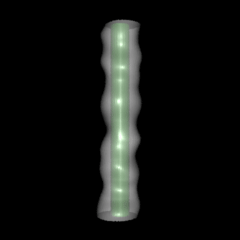Figure 1

#### Layer 2

The purpose of the effect created in this "layer" of the shader is to ramp the bumps so that the base of the cylinder appears smooth and while (simple) turbulance appears to increase with height.

Listing 2

 ```displacement smoke2(float Km = 0.2, freq = 5; string space = "object") { float hump = 0; normal n = normalize(N); /* Layer 1 - moveable bumps */ point pp = transform(space, P); hump = noise(pp * freq); /* Layer 2 - ramp the bumps in 't' */ hump = hump * t; P = P - n * hump * Km; N = calculatenormal(P); } ```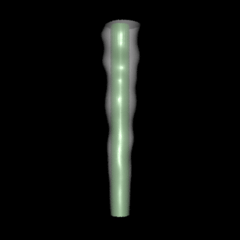Figure 2

#### Layer 3

This layer produces a trumpet shape at the top of the column of "smoke".

Listing 3

 ```displacement smoke3(float Km = 0.2, freq = 5; string space = "object") { float hump = 0; normal n = normalize(N); /* Layer 1 - moveable bumps */ point pp = transform(space, P); hump = noise(pp * freq); /* Layer 2 - ramp the bumps in 't' */ hump = hump * t; /* Layer 3 - trumpet shape */ hump = hump + pow(t, 5); P = P - n * hump * Km; N = calculatenormal(P); }```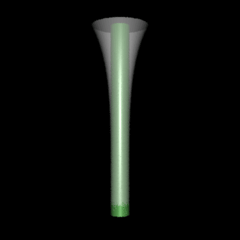Figure 3

#### Layer 4

This layer produces a wave along the x and z axes of the object.

Listing 4

 ```displacement smoke4(float xfreq = 5, zfreq = 3) { float hump = 0; normal n = normalize(N); float x = xcomp(P) + (noise(s * xfreq) - 0.5) * s; float y = ycomp(P); float z = zcomp(P) + (noise(s * xzfreq) - 0.5) * s; P = point(x, y, z); N = calculatenormal(P); } ```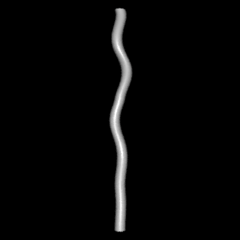Figure 4

#### Combined + Surface

Listing 5

 ```/* Shader description goes here */ displacement smoke4(float Km = 0.2, freq = 5, amp = 1, xfreq = 5, zfreq = 3; string space = "object") { float hump = 0; normal n = normalize(N); /* Layer 1 - moveable bumps */ point pp = transform(space, P); hump = (noise(pp * freq) - 0.5) * amp; /* Layer 2 - ramp the bumps in 't' */ hump = hump * (s); /* Layer 3 - "trumpet" */ hump = hump + pow(s, 5); /* Layer 4 - x,z waviness */ float x = xcomp(P) + (noise(s * xfreq) - 0.5) * s; float y = ycomp(P); float z = zcomp(P) + (noise(s * zfreq) - 0.5) * s; P = point(x, y, z); P = P - n * hump * Km; N = calculatenormal(P); } ```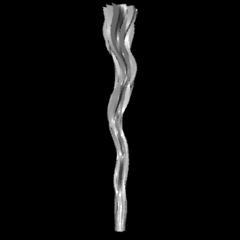Figure 5

Listing 6 (surface shader)

 ```surface rim(float Kd = 1, rim_width = 0.6, Krim = 0.4, flip = 1, fade = 0.5) { normal n = normalize(N); normal nf = faceforward(n, I, n); vector i = normalize(-I); float dot = i.nf; float rw = rim_width + noise(P * 3) * Krim; if(flip) Oi = 1 - smoothstep(rw, 1.0, dot); else Oi = smoothstep(rw, 1.0, dot); Oi = Oi * (1 - pow(s, fade) ) * Os; Ci = Oi * Cs; } ```Figure 6